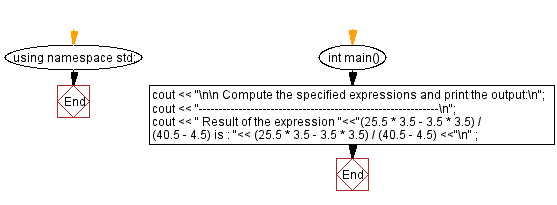﻿ C++ : Compute the specified expressions and print the output

# C++ Exercises: Compute the specified expressions and print the output

## C++ Basic: Exercise-35 with Solution

Write a program in C++ to compute the specified expressions and print the output.

Sample Solution:

C++ Code:

``````#include <iostream>
using namespace std;

int main()
{
cout << "\n\n Compute the specified expressions and print the output:\n";
cout << "------------------------------------------------------------\n";
cout << " Result of the expression "<<"(25.5 * 3.5 - 3.5 * 3.5) / (40.5 - 4.5) is : "<< (25.5 * 3.5 - 3.5 * 3.5) / (40.5 - 4.5) <<"\n" ;
}
``````

Sample Output:

``` Compute the specified expressions and print the output:
------------------------------------------------------------
Result of the expression (25.5 * 3.5 - 3.5 * 3.5) / (40.5 - 4.5) is : 2.13889
```

Flowchart:C++ Code Editor:

What is the difficulty level of this exercise?

﻿

## C++ Programming: Tips of the Day

Why is there no std::stou?

The most pat answer would be that the C library has no corresponding "strtou", and the C++11 string functions are all just thinly veiled wrappers around the C library functions: The std::sto* functions mirror strto*, and the std::to_string functions use sprintf.

Ref: https://bit.ly/3wtz2qA

We are closing our Disqus commenting system for some maintenanace issues. You may write to us at reach[at]yahoo[dot]com or visit us at Facebook